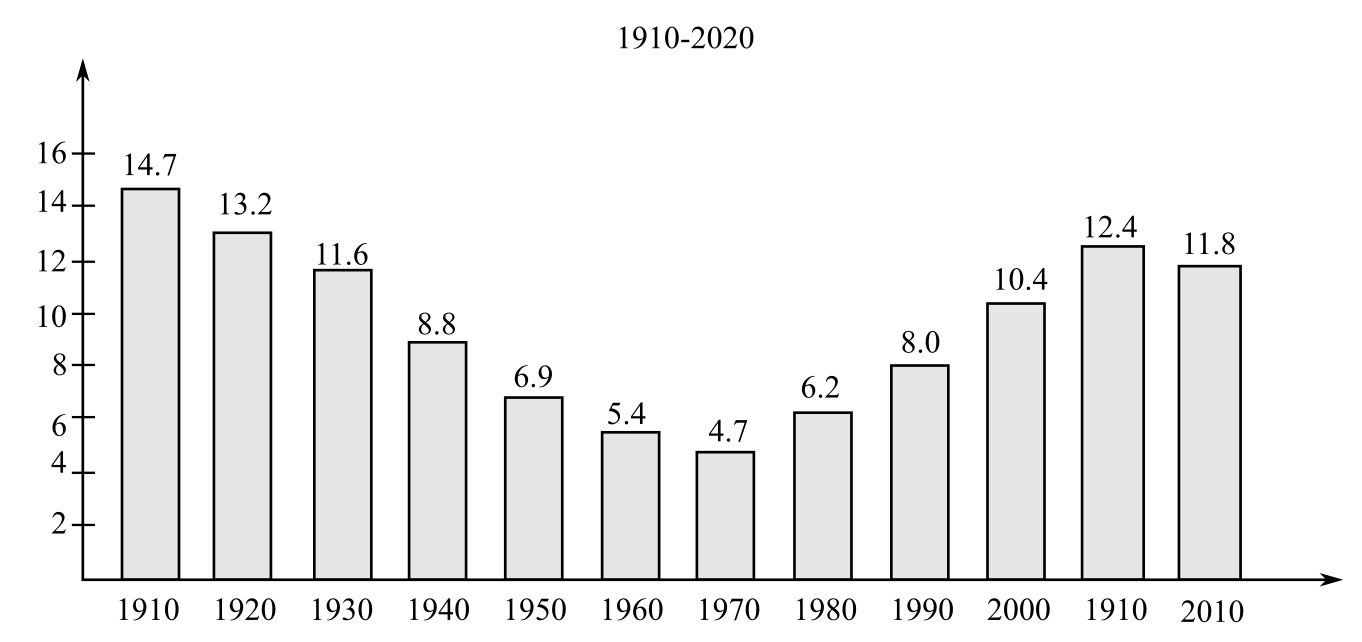Chapter 9.3, Problem 44E### Mathematical Applications for the ...

11th Edition
Ronald J. Harshbarger + 1 other
ISBN: 9781305108042

#### Solutions

Chapter
Section### Mathematical Applications for the ...

11th Edition
Ronald J. Harshbarger + 1 other
ISBN: 9781305108042
Textbook Problem

# b The figure shows the percent of the U.S. population that was foreign-born for selected years from 1910 and projected to 2020.(a) Use the figure to find the average rate of change in the percent of the U.S. population that was foreign- horn from 1960 to 2020. Interpret your result.(b) From the figure, determine for which two consecutive data points the average rate of change in the percent of foreign-born was (i) closest to zero and (ii) furthest from zero.Foreign-Born Population Percent:1910-2020

(a)

To determine

To calculate: The average rate of change in percent of U.S. population from 1960 to 2020. The graph is given as;Explanation

Given Information:

The graph is given as;

Formula used:

If a function f(x) is defined in the interval [a,b], then its average rate of change is given by the formula,

Average rate of change=f(b)f(a)ba

Calculation:

Consider the provided statement,

The graph is given as

(b)

To determine

To calculate: The consecutive data points for which the average rate of change in percent of U.S. population is closest and furthest from zero. The graph is given as### Still sussing out bartleby?

Check out a sample textbook solution.

See a sample solution

#### The Solution to Your Study Problems

Bartleby provides explanations to thousands of textbook problems written by our experts, many with advanced degrees!

Get Started

#### Differentiate the function. g(x)=74x23x+12

Single Variable Calculus: Early Transcendentals, Volume I

#### The third partial sum of is:

Study Guide for Stewart's Multivariable Calculus, 8th

#### The average value of f(x) = 3x2 + 1 on the interval [2, 4] is: a) 29 b) 66 c) 58 d) 36

Study Guide for Stewart's Single Variable Calculus: Early Transcendentals, 8th